﻿ Design of Refractive Index Profile for Multimode Optical Fibers with Low Differential Mode Delay

### Design of Refractive Index Profile for Multimode Optical Fibers with Low Differential Mode Delay

Anton V. BourdineOPEN ACCESSPEER-REVIEWED

## Design of Refractive Index Profile for Multimode Optical Fibers with Low Differential Mode Delay

Anton V. BourdineDept. of Communications Lines, Povolzhskiy State University of Telecommunications and Informatics (PSUTI), Samara, Russia

### Abstract

This work presents an alternative method for design of refractive index profile for silica GeO2-doped graded-index multimode optical fibers 50/125 with low differential mode delay (DMD), providing equalization between group velocities of selected guided modes. Here the objective function is represented as a sum of squares of deviations between points of current iteration group velocity map, which has been calculated over modes with particular orders, and certain reference group velocity value. During the objective function formation it is proposed to take into account only selected guided modes, that depend on the type of laser source and launch conditions. In the given article we represent some results of introduced method approbation with following simulation of fiber optic link based on proposed multimode fibers with low DMD under the core geometry variations.

### At a glance: Figures

123
Prev Next

• Bourdine, Anton V.. "Design of Refractive Index Profile for Multimode Optical Fibers with Low Differential Mode Delay." Journal of Optoelectronics Engineering 1.1 (2013): 5-13.
• Bourdine, A. V. (2013). Design of Refractive Index Profile for Multimode Optical Fibers with Low Differential Mode Delay. Journal of Optoelectronics Engineering, 1(1), 5-13.
• Bourdine, Anton V.. "Design of Refractive Index Profile for Multimode Optical Fibers with Low Differential Mode Delay." Journal of Optoelectronics Engineering 1, no. 1 (2013): 5-13.

 Import into BibTeX Import into EndNote Import into RefMan Import into RefWorks

### 1. Introduction

Differential mode delay (DMD) is the main factor of pulse distortion for laser-based multi-Gigabit data transmission over conventional silica multimode graded-index fibers of both OM1/OM2 and modern OM3/OM4 categories . It is an effect caused by narrow laser beam injection into the center region of multimode fiber core. As emission from the conventional VCSEL usually consists of about 5 or 6 transversal modes LPlm with maximal azimuthal order l not more than 3, and single-mode Fabry-Perot laser diodes injects just fundamental mode LP01, only several guided modes are excited in multimode fiber [2, 4], and optical signal propagates over multimode fiber link in a so-called few-mode regime. DMD produces the jitter that dramatically grows up under fiber length increase. It appears as output pulse splitting, and distortion pattern depends on parameters of particular laser-source/multimode fiber couple under the specific launch conditions.

Nowadays a new generation of graded-index multimode fibers is specially developed for laser-based high bit rate data transmission. This type of multimode fibers is known as laser-optimized fibers (LOMFs) and have been subsumed to OM3 and OM4 categories. LOMFs differ from the first generation multimode fibers of the OM1/OM2 categories by the almost ideal graded refractive index power-law α-profile without great central defect and strong refractive index fluctuations. Many well-known fiber manufactures produce commercial trade-marked LOMF families, developed for both short and long wavelength specifications λ=850nm and λ=1310nm , [5, 6]. The most top applications of LOMFs are associated with modern data center cabling systems, high bit rate SANs and LANs.

However DMD occurs even in the LOMFs, and it can become critical during the passage to higher bit rates. Although there are VCSEL modulation limits, DMD is also one of the main reasons for using the parallel-optics transmission instead of serial transmission in IEEE 802.3ba standard of 40/100G, which has been ratified in June 2010. Here the maximal multimode fiber link length is strongly limited down to 100m for OM3 and 150m for OM4 by IEEE 802.3ba specifications 40GBase-SR4 and 100GBase-SR10. Nevertheless, in practice, there are different examples of in-premises networks with both backbones and horizontal fiber cabling systems based on multimode fibers requiring link length extension comparing to distances limited by IEEE specifications.

Here we introduce an alternative graded-index multimode fiber with low DMD, providing a serial 40/100G data transmission over enhanced distance without pulse splitting due to DMD. We also present the method for its design. Some results of proposed method approbation for profile synthesis of mentioned silica GeO2-doped fibers 50/125 are represented.

### 2. Review of Multimode Fibers with Enhanced Bandwidth

Nowadays we know a lot of methods for design of multimode fibers with enhanced bandwidth. They have been developed and assigned for the few-mode regime. The most of them are based on DMD monitoring during the fiber preform manufacturing.

Thus refractive index profile of multimode fiber  is defined over several iterations by comparing results of DMD measurements produced for two fiber samples, which have been drawn from the current preform and previous one, before MCVD assembly program correction had been carried out. However, this multistage empirical correction of fiber preform parameters, based on results of previous iteration DMD measurements uses fiber performs, which have been designed initially for multimode regime.

In [8, 9] the process of fiber manufacturing includes the following steps. Preliminary a set of conventional multimode fibers are tested, DMD profiles are plot, and fiber samples with low DMD are identified. Then DMD profiles of selected fibers are superimposed, and after the averaging an optimal DMD profile is obtained. As a result it is possible to pass to an optimal refractive index profile. But these DMD profiles were selected as the best plots with minimal DMD, which have been measured on conventional multimode fibers of the 1st and 2nd generations.

In  DMD is monitored by previous iteration preform testing, and the optimal refractive index profile of the current iteration preform is formed by layer-to-layer correction according to results of the optimal DMD profile calculation. But here a method for coupling between profile α-parameter and DMD value at the particular radial coordinate is applied and based on an integral DMD estimation for all guided modes supported by this fiber.

The main aim of the method  is to obtain a LOMF, which will have low DMD both for λ=850nm and λ=1310nm. It is based on computing of DMD maps (DMD value distribution over principal mode numbers) of LOMF model refractive index profiles optimized for data transmission either under λ=850nm or λ=1310nm, and following DMD map updates with refractive index profile recalculation to provide low DMD for both wavelengths. Here during computing an overfilled launching of laser source excitation is considered. Therefore this refractive index profile can not be optimal for central launching, which is one of the well-known methods for multimode link bandwidth enhancing under the few-mode regime .

Therefore the following main factors should be taken into account during the design of the graded-index refractive index profiles providing low DMD to obtain the best results for the few-mode regime: 1) LOMF geometry parameters and refractive index profile should be used as reference data; 2) optimal refractive index profile design, reference profile modification or injecting of special elements to the base fiber construction should be referred to corresponding DMD map or DMD profile computed for selected guided modes; 3) the same actions should be carried out, taking into account the type of laser source and launch conditions.

### 3. Multimode Fiber Model

3.1. Theory

The general design of proposed multimode fiber with low DMD is similar to the conventional graded-index multimode fiber 50/125: it comprises a core with diameter 50 μm surrounded by outer cladding with refractive index nN. Here DMD is reduced by special refractive index profile form in the core region, which provides minimization of some value F described by following expression:(1)

where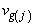is group velocity of j-th guided mode LPlm(j) propagating over proposed fiber;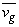is reference group velocity, it is applied for group velocity map equalization; M is a total number of mode components transferring an excited by laser source few-mode signal with normalized amplitude not the less then Aj> 0.1 and mode transferred over fiber core (it is also known as optical confinement factor) is not the less then. M depends on the following factors: 1) launch conditions and 2) transversal modes of emission at the laser output – this is defined by the type of laser source – VCSEL or singlemode laser (e.g. Fabry-Perot laser). In general case, M is less than 40 if we take into account features of the few-mode regime.

Therefore the desired refractive index profile is obtained by direct minimization of the proposed objective function F (1). Here arguments of objective function are the elements of array of profile parameters hk that completely describe fiber refractive index profile, represented by the set of N points: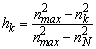(2)

where nk is refractive index of k layer (k=0…N); nmax is the maximal core refractive index.

Profile optimization leads to minimization of the objective function with great number of arguments, and simplex search method of Nelder and Mead can be applied, that can be submitted by [13, 4] devoted to problems of the optical waveguide synthesis with particular parameters and characteristics.

Accuracy of mode group velocity calculation depends on the number of elements in profile parameter array. Enhancing of the total number of profile points in [hk] will greatly increase the accuracy under computing time expanding. However, as a result of minimization of the objective function (1) with great number of arguments, a refractive index profile will be obtained that would not be realized in practice. Therefore the number of arguments or profile points should provide both high accuracy of calculations under acceptable computing time and following realization ability of designed refractive index profile for multimode fiber with low DMD.

Proposed design of multimode fibers with low DMD includes the following steps. Preliminary, a reference valueof mode group velocity should be defined. Initial profile at the first iteration of optimization can be approximated by conventional smoothed power-law α-profile, which is represented by the set [hk] with N elements.

It is obvious that the described algorithm requires fast and simple method for analysis of the optical fibers with an arbitrary profile, which provides estimation of parameters not only for fundamental but also high-order modes. We introduced an extension of modified Gaussian approximation (EMGA) generalized for estimation of any order guided mode parameters, propagating along weakly guiding optical fibers with an arbitrary axial-symmetric refractive index profiles . This method is based on stratification and Gaussian approximation methods, and it permits to decrease the computing time much, especially for the higher-order mode parameter calculations under acceptable accuracy by making a passage to analytical expressions both for the variational expression and characteristic equation, and for the first and second derivatives of propagation constant . It is proposed to apply this fast and simple approximate method for multiple solution of direct problem of multimode fiber analysis during the refractive profile synthesis by the objective function F minimization.

3.2. Extension of Modified Gaussian Approximation

EMGA is based on classical Gaussian approximation  of radial mode field distribution Fm(l)(R) in the weakly guiding optical waveguide with an arbitrary refractive index profile by the well-known Laguerre-Gauss function expression [1, 17, 18], describing a mode field distribution in weakly guiding optical waveguide with ideal infinite parabolic index profile: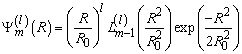(3)

where l and m are azimuth and radial orders of mode LPlm;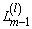is Laguerre polynomial.

EMGA leads to equivalent normalized mode field radius R0 estimation by solving a characteristic equation, which is derived from propagation constant variational expression under following passage to square core mode parameter U2 variational expression, written for investigated weakly guiding optical waveguide with given refractive index profile. Parameter R0 is basic for this method and completely defines mode transmission parameters.

Unlike the well known methods and their modifications Unlike the well known methods and their modifications [19-25], based on conventional Gaussian approximation, in EMGA optical fiber with an arbitrary graded axial-symmetric index profile is considered as a multi-cladding optical fiber. Therefore, core region refractive index profile can be represented in the form of the set of N layers in which the refractive index stays a constant on [15, 16]: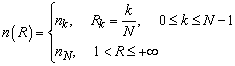(4)

and any profile function f(R) related with refractive index profile n(R) as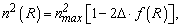where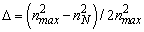is profile height parameter, can be written in the following form based on parameter hk: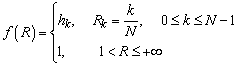(5)

The refractive index profile representation described above permits to write the variational expression for core mode parameterand then the characteristic equation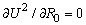in the analytical form of the finite nested sums as following , :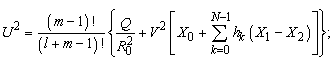(6)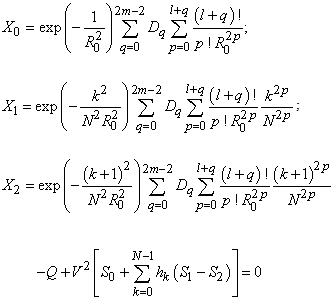(7);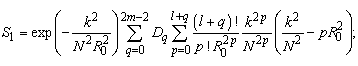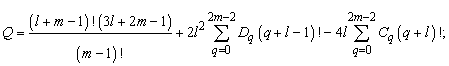where(8a)(8b)

andis coefficient polynomial representation in the form of power series [26, 27]:(9a)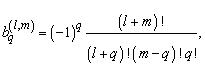(9b)is normalized frequency;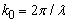is wavenumber; λ is wavelength.

Therefore analysis of weakly guiding single-cladding optical fiber leads to following. Refractive index profile is represented by the profile function (5) in the form of N layers. Fiber parameters and mode orders l and m are substituted to the characteristic equation (7). By means of numerical solution (7), the normalized equivalent mode field radius R0 will be obtained. Then R0 is substituted to the expression (6), and mode core parameter U is estimated, that permits the evaluation of propagation constant β for guided mode LPlm by the well known expression [1,17,18]:(10)

Solution of the characteristic equation (12) is correct under V>1, and it should satisfy the guided mode cutoff condition [17,18]: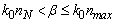(11)

Optical confinement factor Pco can be considered as the second criterion for identification of phantom solution: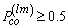(12)

If we take into account Gaussian approximation, parameter Pco is defined by analytical expression derived in  from the generalized integral form for weakly guiding optical fibers presented in :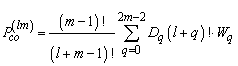(13)

where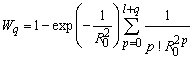3.3. Mode Group Velocity

Realization of introduced method for design of graded-index refractive profile for multimode fibers with low DMD also requires a passage to analytical expression for mode group velocity. Guided mode group velocity vg is related to propagation constant β by the well known formula [1,17,18]: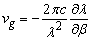(14)

Therefore by differentiating of expression (10) with respect to wavelength under taking into account simple ratio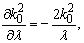first partial derivative of the square of propagation constant will be obtained: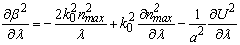(15)

By substituting expressions (15) to the formula (14) and taking into account simple relation proposed in :expression for the group velocity can be rewritten in the following form:(16)

By differentiating expression (6) and (7) with respect to wavelength, first derivative of square of core mode parameter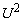and normalized equivalent mode field radius R0 will be obtained: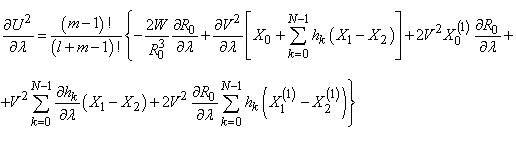(17)(18)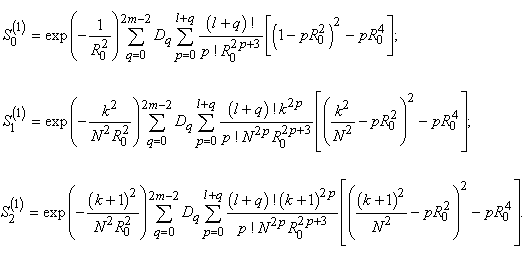3.4. Material Dispersion

Here Sellmeier equation is used to take into account the refractive index dependence on wavelength [1,17,18]: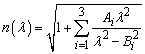(19)

where Ai and Bi are Sellmeier coefficients (Bi is also denoted as the resonance wavelength) which were empirically measured for GeO2-doped glasses [29, 30] for the particular dopant concentration. Here the method, described in  is applied to estimate Sellemier coefficients at the indefinite graded-index refractive index profile points.

A passage from the differentiation operator to its square is applied analogously to propagation constant derivatives. It is based on the following relation proposed in :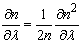(20)

This passage simplifies expressions for the first order derivative of the refractive index, which have the following form: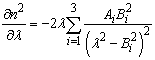(21)

Therefore the first order derivative of the profile height parameter can be expressed as follows: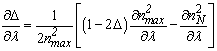(22)

Derivative of profile parameter hk defined by formula (2) is determined by following expression: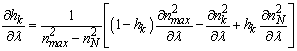(23)

Finally, by applying the relation (21), the first order derivative of the normalized frequency can be expressed by the same derivatives of V2:(24)

### 4. Results

4.1. Results of Profile Synthesis

As it was supposed above, the designed fiber preform with synthesized profile will be manufactured by conventional MCVD or PCVD process, a real LOMF Corning® sample of OM2+/OM3 category was used as a reference fiber. Refractive index profile of this sample was measured by the fiber analyzer EXFO NR-9200HR by means of refracted near-field method , and its reconstruction based on measurement results is shown on Figure 1. Here profile form seems to be almost power-law without great defects at the core center and steps at the core/cladding boundary (Figure 1(a)). However some local refractive index fluctuations should be noted (Figure 1(b)).

By applying the EMGA for analysis of reference fiber, a set of guided mode group velocities can be computed at the particular wavelength. According to proposed method the total number M of guided modes should be taken into account during the group velocity map constructing. It depends on the type of laser and launching conditions. Thus, conventional VCSEL emits the following six transversal modes LP01, LP02, LP11, LP12, LP21, LP31 under the operating wavelength λ=850nm [2, 3, 4]. By applying a well known analytical expression for mode coupling coefficients at the centralized connection of diverse type fibers without misalignments, derived in  by conventional overlap integral method under radial mode field representation in the terms of Gaussian approximation: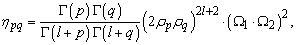(25)

where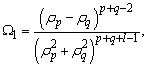p and q are radial orders of launched and excited modes;

ρp and ρq are mode field radiuses; Γ is gamma function.

The total number of excited modes M in reference fiber can be estimated under inequations mentioned above Aj>0.1 and.

Figure 1. Reference refractive index profile of real fiber sample LOMF Corning OM2+/OM3: (a) full profile; (b) local profile fragment around core center
Figure 2. Refractive index profile of multimode fiber with low DMD, optimized for operation under VCSEL (λ=850nm) excitation with central launch

Thus, during the centralized launching, only M=12 guided modes LPlm (l=0.3; m=1.3) should be considered due to negligible amplitudes of the higher-order modes with radial orders m>3, and array of mode group velocity with mentioned order was formed. First the minimal, maximal and mean values ofwere estimated and considered as reference group velocities. Further on, a whole interval from 2.02340105 to 2.02411105 km/s have been divided on 40 values, and each value was applied as referencefor profile synthesis. The best result corresponding to the minimal deviations of DMD map was obtained for 36-th value from investigated interval under=2.02404105km/s. Here refractive index profile of multimode fiber with low DMD has been synthesized under mentioned conditions. It is shown on Figure 2. It is also the simplest in comparison with other computed profiles providing low DMD.

4.2.Model for Link with Irregular Multimode Optical Fiber with Low DMD

In practice, different irregularities occur in real fibers due to techniques features of preform manufacturing and fiber drawing, which should be taken into account during the modeling of signal propagation process. First of all, it is longitudinal core radius variation. Also some local profile distortions can be noted in spite of features of modern MCVD or PCVD technologies providing a fiber preform profile reconstruction with high accuracy. There are some faint local refractive index fluctuations even for the last generation LOMFs (see Figure 2(b)) . Therefore, these two factors should be modeled to simulate proposed multimode fiber with low DMD closer to the real prototype.

According to , variations of core geometrical parameters are described well enough by normal probability law even for the first generation graded fibers. In spite of the fact that the fiber draw speed is about 1.2m/s , it is proposed to consider the worst case with core variation length period of 2.3m. Parameters of normal probability law can be identified by conventional multimode fiber specifications [5, 6] core diameter is 50±3μm. Therefore, the mean value of core radius is 25μm, while the mean-squared deviation is 0.5μm according to three-sigma rule.

Figure 3. Distorted refractive index profile of multimode fiber with low DMD: (a) full profile; (b) local profile fragment around core center

The same approach was applied to simulate profile distortions. As we consider multimode fiber link with short length, it is supposed that the general profile form stays constant along the fiber. Local refractive index fluctuations were added to synthesized profile form (Figure 2), set by 250 points as well as by normal probability law. Here the local mean value corresponds the refractive index nk at the point with radial coordinate rk, while the mean-squared deviation of n was estimated by distortions of reference profile, reconstructed from the data of real LOMF profile measurements (Figure 1(b)) and also by three-sigma ruleas a result this parameter is 5•10-5. Distorted graded refractive index profile and its scaled fragment at the core center of the proposed multimode fiber with low DMD are represented on Figure 3 (a) and (b) respectively.

Having been introduced in early works [35, 36] the piece-wise representation (Figure 4) of multimode fiber link would be applied to simulate a process of mode interaction and mixing due to core diameter variation during a few-mode signal propagation over multimode fiber. We consider single silica weakly guiding circular multimode fibers with the axial symmetric refractive index profile, limited by single outer cladding. Boundaries of regular spans are represented as ideal axially alignment splice of two almost similar optical waveguides with mismatching parameters

The fiber is divided into regular spans. Inside of the span waveguide parameters are considered as constant. It is supposed that each guided mode have been excited by laser source (or impinging on span boundary) transfers an optical pulse with Gaussian form. Amplitudes of signal mode components decrease proportionally to attenuation, mentioned in fiber specification, and mode core power parameter Pco. It is supposed that inside of the regular span there are no any mode interactions and mixing. The signal is distorted due to difference between group velocities and amplitudes of modes, or by DMD effect respectively. Besides, we observe pulse spreading transferred by each mode. It happens due to chromatic dispersion also estimated by EMGA [15, 16]. Power redistribution between the signal modal components, caused by irregular periodical structure of investigated fiber, is taken into account by estimation of mode coupling coefficients (25) at the joints of regular spans. Here only guided modes are considered as the main issue under pulse dynamics investigation during propagation over described multimode fiber core diameter variations. However, in fact, power loss due to component transformation from guided to leaky mode, and reflections are taken into account by mode coupling calculation at the boundaries of regular spans.

4.3. Simulation Results

First of all, a simplified time-domain model [35, 36] of fiber optic transmission link was applied for preliminary estimation of the obtained profile effectiveness for a few-mode 10 Gb/s (full width at a half maximum (FWHM) t05=90.9 ps) optical pulse serial transmission. Here only DMD was taken into account, and mode interaction due to real fiber irregularity was neglected. According to computed results, pulse splitting due to DMD did not occur for the distance Lmax=1.5km and even more, instead 10Gbase-SR limit for the OM4 Cat. LOMF Lmax=600m. As conventional in-premises network link length is less then 1km , there is no point in doing the following simulation for enchanced distance under the 10Gb/s bit rate: pulse will keep its envelope, FWHM will not increase more then by 3.5 times, and well known electronic dispersion compensation technique (EDC) can be applied for signal compressing as required.

Therefore, the following simulations were produced for the 40 Gb/s bit rate serial data transmission corresponding to FWHM t05=22.7ps . Based on the mentioned simplified model for a “regular” fiber, a pulse dynamics was computed, and estimated maximal link length Lmax was defined, where pulse splitting due to DMD did not occur or/and pulse dispersion was not more than 16.5 ps  corresponding to BER=10-15. Distorted pulse at the output of 300m of multimode optical fiber with refractive index profile, shown on Figure 2, has been computed for VCSEL-based transceiver under centralized launching. It is shown on Figure 5. Pulse did not split and kept its envelope, and estimated dispersion was D=30.63 ps at the receiver end.

Figure 5. Input pulse at the transmitter end (dash-dotted line) and output distorted pulse at the receiver end (solid line) under the “regular”fiber length L=300m

Results of the following computing are shown, the proposed refractive index profile (Figure 2) provides a 40 Gb/s serial data transmission under dispersion less then desired 16.5 ps under the fiber length down to 160m.

Then, according to time-domain model, described above, a few-mode signal dynamics computation during propagation over “irregular” multimode fiber with low DMD under core radius variation was produced. Here refractive index profile distortions were also taken into account: profile sample presented on Figure 3 was considered. A propagation of Gaussian pulse with FWHM t05=22.7ps (40Gb/s), excited by VCSEL (λ=850nm, spectral width Δλ=1nm) generating optical signal with six transversal modes under centralized launching over multimode fiber with low DMD (Figure 3) was considered. Sample of core radius distribution along investigated fiber link is shown on Figure 6. The length of regular span was chosen to be equal to 3m (Lr=3m).

Figure 7. Gaussian pulse dynamics with initial width t05=22.7ps during propagation over of introduced multimode fiber with low DMD under VCSEL excitation, centralized launching, core radius variations and profile distortions

A few-mode signal dynamics diagram, computed under mentioned conditions is shown on Figure 7. Calculated pulse forms at the transmitter and receiver ends also for link lengths 300m and 160m as in the case of model regular fiber with smoothed graded refractive index profile are presented on Figure 8 (a) and (b).

In spite of the fact that longitudinal core radius variation and refractive index distortions were added to initial synthesized profile of multimode fiber with low DMD, pulse also did not split and kept its envelope. Moreover it is clearer to Gaussian form in comparison with pulse at the output of “regular” model fiber. This fact can be explained by mode mixing and power diffusion processes. Signal dispersion both in the case of irregular multimode fiber with distorted profile and in the case of model regular multimode fiber is the same order.

According to computed results, proposed multimode fiber with low DMD provided by synthesized special form of refractive index profile supports the 40Gb/s bit rate serial data transmission up to distanse Lmax=160m without any additional actions for pulse compression instead ratified by 40Gbase-SR4 limit for the OM4 Cat. LOMF with approximately the same length Lmax=150m and required parallel data transmission 4x10Gb/s.

### 5. Conclusion

This work presents the alternative method for design of refractive index profile for multimode optical fibers with low DMD. It differs from well kown methods by following factors: 1) LOMF geometry parameters, refractive index profile and group velocity diagram are applied as reference data; 2) optimal refractive index profile design is based on object function (1) minimization referred the selcted mode group velocity diagram deviations from the reference value.

Results of proposed method approbation for profile synthesis of silica GeO2-doped graded-index multimode fibers 50/125 during VCSEL excitation under generated six transversal mode optical signal and centralized launching both for “regular” and “irreglar” multimode fibers with core radius variation and adding of distortions to refractive index profile are represented. Simulation of fiber irregularity and local profile deviations from the optimal form was based on normal probability law, which parameters were estimated by specifications and data of measurements of real fiber samples.

Figure 8. Input pulse at the transmitter end (dash-dotted line) and output distorted pulse at the receiver end (solid line) under the “irregular”fiber length: (a) L=300m; (b) 160m

Analysis of obtained results shows the effectiveness of proposed method for design multimode fibers with improved bandwidth, provided by special refractive index profile, which is optimal for a few-mode regime of multi-Gigabit data transmission. Thus, according to computed results, proposed multimode fiber provides VCSEL-based 40Gb/s bit rate serial data transmission up to distanse Lmax=160m without any additional actions for pulse compression instead being ratified by 40Gbase-SR4 limit for the OM4 Cat. LOMF with approximately the same length Lmax=150m and required parallel data transmission 4x10Gb/s.

Moreover, by using proposed fibers, it is possible to enhance greatly the maximal distance for a few-mode signal with initial FWHM t05=22.7ps (40Gb/s) without DMD distortions. As a pulse keeps its envelope during propagation along proposed fiber, but extends due to high chromatic dispersion under the wavelength λ=850nm, a combined application together with well known EDC technique will provide more bandwidth enhancement.

However, the following research work is required: mode mixing and power diffusion process due to micro/macro bends and tension of the real installed optical cables should be taken into account. Also a refractive profile optimization under the less core diameter or together with core diameter as an addition argument of the objective function can reduce the number of mode components tnsferring the optical signal. Finally, a development of algorithm for selection of reference mode group velocity and reference refractive index profile is required, that will make it possible to obtain more effective design results.

### References

  Bottacchi S., Multi-Gigabit transmission over multimode optical fibre. Theory and design methods for 10GbE systems, John Wiley Sons Ltd, West Sussex, 2006.In article CrossRef PubMed  Degen Ch., Elsaber W., Fischer I., “Transverse modes in oxide confined VCSELsinfluence of pump profile, spatial hole burning, and thermal effects”, Optics Express, 5(3), 38-47. 1999.In article CrossRef PubMed  Zei L.-G., Ebers S., Kropp J.-R., Petermann K., “Noise performance of multimode VCSELs”, IEEE Journal of Lightwave Technology, 19(6), 884-892. 2001.In article CrossRef  Gholami A., Toffano Z., Destrez A., Pez M., Quentel F., “Spatiotemporal and thermal analysis of VCSEL for short-range Gigabit optical links”, Journal of Optical and Quantum Electronics, 38(4-6), 479-493. 2006.In article CrossRef  Semenov A.B., Fiber optic subsystems of the modern SCS, DMK Press, Moscow, 2007.In article PubMed  Listvin A., Listvin V., Shvirkov D., Optical fibers for telecommunication lines, LESARart, Moscow, 2003.In article  Buckler M.J., Kummer R., Mettler S.C., Miller M., “Fabrication of optical fibers using differential mode-group delay measurement”, Patent US 4286979. 1981.In article  Abbot J.S., Harshbarger D.E., “A multimode fiber and method for forming it”, Patent WO 00/50936. 2000.In article  Abbot J.S., Harsbarger D.E., “Laser optimized multimode fiber and method for use with laser and system employing same”, Patent US 2002/0197038. 2002.In article  Bangalore Krishnaswamy P., Dutta S., Panneerselvam S.R., Nageswaran S.K., “Optical fiber having higher bandwidth and method for producing the same”, Patent WO 2007/043060. 2007.In article  Golowich S.E., Jones S.L., Ritger A.J., Thornburg S., “Apparatus and method for improving bandwidth of multimode fibers”, Patent US 6574403. 2003.In article  Freund R.E., Bunge Ch.-A., Ledentsov N.N., Molin D., Caspar Ch., “High-speed transmission in multimode fibers”, IEEE Journal of Lightwave Technology, 28(4), 569-586. 2010.In article CrossRef  Bogolyubov A.N., Krasilnikov A.V., Minayev D.V., Sveshnikov A.G., “Finite difference method for solution of the problem of waveguide system synthesis”, Mathematical Modeling, 12(1), 13-24. 2000.In article  Bogolyubov A.N., Butkarev I.A., Sveshnikov A.G., “Synthesis of optical fibers”, Radiotechnika, 12, 4-12. 2004.In article  Bourdine A.V., “Method for chromatic dispersion estimation of high-order guided modes in graded index single-cladding fibers”, Proceedings of SPIE, 6605, 660509-1 660509-13. 2006.In article  Bourdine A.V., Delmukhametov O.R., “Calculation of higher order guided mode propagation parameters based on combination of modified Gaussian approximation and finite element method”, Telecommunications, 9, 33-40. 2010.In article  Snyder A., Love J., Optical waveguide theory, Chapman Hall, London, 1983.In article  Adams M.J., An introduction to optical waveguides, John Wiley Sons Ltd, New York, 1981.In article  Sharma A., Hosain S.I., Ghatak A.K., “The fundamental mode of graded-index fibressimple and accurate variational methods”, Optical and Quantum Electronics, 14(1), 7-15. 1982.In article CrossRef  Tewari R., Hosain S.I., Thyagarajan K., “Scalar variational analysis of single mode fibers with Gaussian and smoothed-out profiles”, Optics Communications, 48(3), 176-180. 1983.In article CrossRef  Oksanen M.I., Lindell I.V., “Variational analysis of anisotropic graded-index optical fibers”, IEEE Journal of Lightwave Technology, 7(1), 87-91. 1989.In article CrossRef  Ankiewicz A., Peng G.-D., “Generalized Gaussian approximation for single-mode fibers”, IEEE Journal of Lightwave Technology, 10(1), 22-27. 1992.In article CrossRef  Holmes M.J., Spirit D.M., Payne F.P., “New Gaussian-based approximation for modeling non-linear effects in optical fibers”, IEEE Journal of Lightwave Technology, 12(2), 193-201. 1994.In article CrossRef  Wu M.-Sh., Lee M.-H., Tsai W.-H., “Variational analysis of single-mode graded-core W-fibers”, IEEE Journal of Lightwave Technology, 14(1), 121-125. 1996.In article CrossRef  Meher H., Hosain S.I., “Variational approximations for single-mode graded-index fiberssome interesting applications”, Journal of Optical Communications, 24(1), 25-30. 2003.In article CrossRef  Gradstein I., Ryjik I., Tables of integrals, GIFML, Moscow, 1963.In article  Abramovitz M., Stegan I., Handbook of Mathematical Functions with Formulas, Graphs and Mathematical Tables, Nauka, Moscow, 1979.In article  Burdin V.A., Andreev R.V., .Praporschikov D.E, “Model and algorithm of optimization of a refractive index profile of single-mode fibers for optical communication networks”, Proceedings of SPIE, 5485, 63-74. 2003.In article  Fleming J.W., “Dispersion in GeO2-SiO2 glasses”, Applied Optics, 23(24), 4886 - 4493. 1984.In article CrossRef  Binh L.N., Design guidelines for ultra-broadmand dispersion-flattened optical fibers with segmented-core index profile, Technical Report MECSE-14-2003, Monash University, Clayton, 2003.In article  Burdin V.A., “Methods for computation of Sellmeier coefficients for dispersion analysis of silica optical fibers”, Infocommunication Technologies, 4(2), 3-34. 2006.In article  Bourdine A.V., Yablochkin K.A., “Research of refractive index profile defects of silica graded-index multimode fibers of telecommunication cables”, Infocomminication Technologies, 8(2), 22-27. 2010.In article  Srapionov V.A., “Mode coupling at the splices of optical fibers with mismatched parameters”, Elektrosvyaz, 10, 10-12. 1985.In article  Krawarik P., Watkins L., “Fiber geometry specifications and its relations to measured fiber statistics”, Applied Optics, 17(24), 3984-3989. 1978.In article CrossRef PubMed  Bourdine A.V., “Simulation results of few-mode signal propagation over graded multimode optical fibers with periodical slowly varying core diameter”, Proceedings of SPIE 7374, 737406-01-737406-11. 2009.In article  Bourdine A.V., Delmukhametov O.R., Makarov V.S., Yablochkin K.A., “Multimode optical fiber core deviation influence on signal propagation under few-mode conditions”, Infocommunication Technologies, 8(3), 12-21. 2010.In article  Cunningham D., Nowell M., Hanson D., Kazovsky L., “The IEEE 802.3z worst case link model for optical physical media dependent specification”, IEEE 802.3z Task Force Presentation materials, 2. 1998.In article